# GSEB Solutions Class 12 Maths Chapter 7 Integrals Ex 7.6

Gujarat Board GSEB Textbook Solutions Class 12 Maths Chapter 7 Integrals Ex 7.6 Textbook Questions and Answers.

## Gujarat Board Textbook Solutions Class 11 Maths Chapter 7 Integrals Ex 7.6

Integrate the following functions:
Question 1.
x sin x
Solution:
Let I = ∫x sin x dx
We know that ∫uv dx = u∫v dx – ∫(u’ ∫v dx)dx
Put u = x and v = sin x.
∴ I = x ∫sin x dx = ∫[($$\frac{d}{dx}$$ x) ∫sin x dx]dx
= – x cos x – ∫1. (- cos x)dx
= – x cos x + ∫cos x dx = – x cos x + sin x + C.Question 2.
x sin 3x
Solution:
Let I = ∫xsin 3x dx. Taking u = x and v = sin 3x.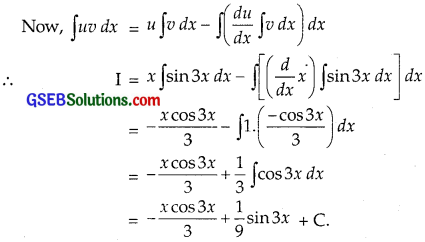Question 3.
x2ex
Solution:
Let I = ∫x2ex dx, Put u = x2 and v = exdx.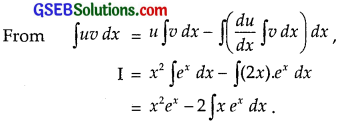Taking x as I function, integrating again, we get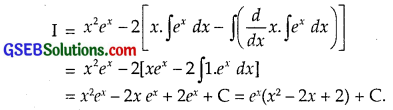Question 4.
x log x
Solution:
Let I = ∫xlog x dx, Put u = log x and v = x.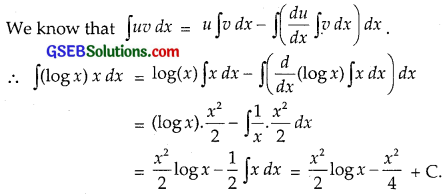Question 5.
x log 2x
Solution:
Let I = ∫xlog 2x dx = ∫(log 2x).x dx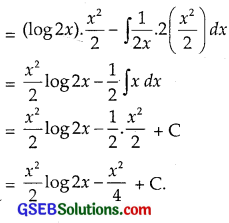Question 6.
x2log x
Solution: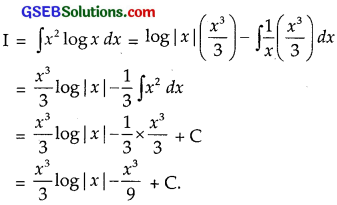Question 7.
x sin-1x
Solution: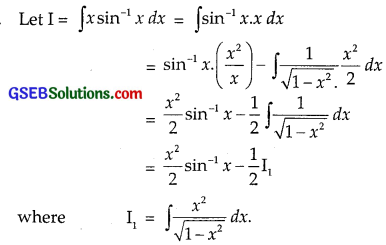Put x = sinθ so that dx = cosθdθ.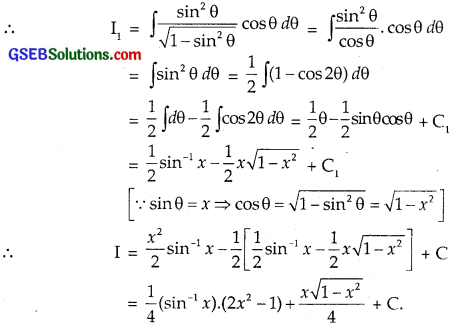Question 8.
x tan-1x
Solution:
Let I = ∫x tan-1 x dx. Put x = tan-1x and v = x.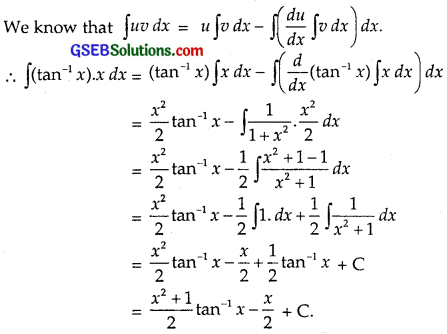Question 9.
x cos-1x
Solution:
Let I = ∫xcos-1 x dx = ∫cos-1 x.x dx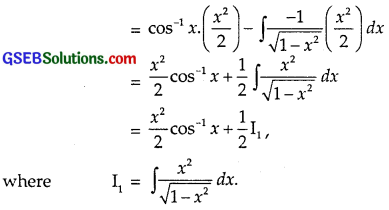Putting x = cosθ so that dx = – sinθ dθ.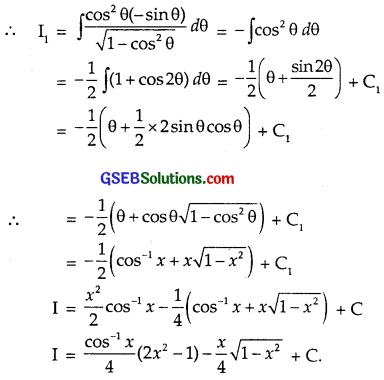Question 10.
(sin-1x)2
Solution:
Let I = ∫(sin-1 x2)dx.
Put sin-1x = θ ⇒ x = sin θ ⇒ dx = cosθ dθ.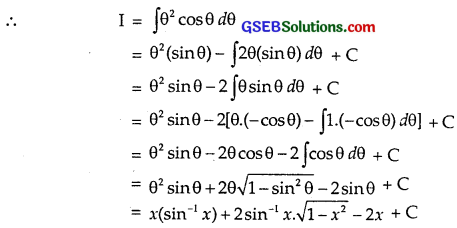Question 11.
$$\frac{x \cos ^{-1} x}{\sqrt{1-x^{2}}}$$
Solution: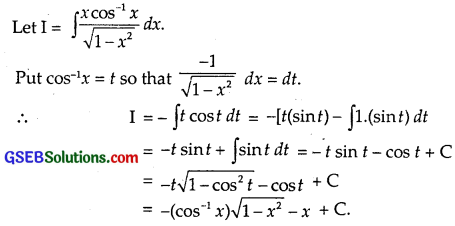Question 12.
x sec2x
Solution: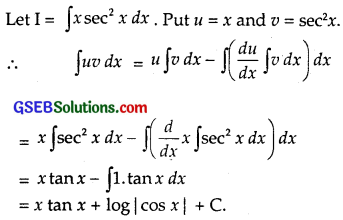Question 13.
tan-1x
Solution: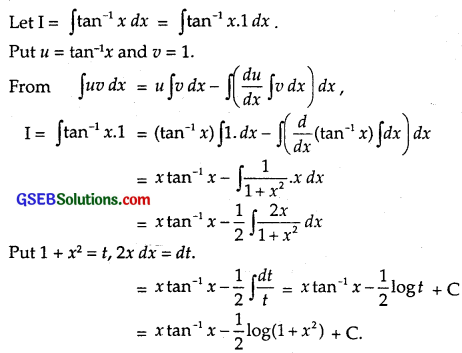Question 14.
x(log x)2
Solution: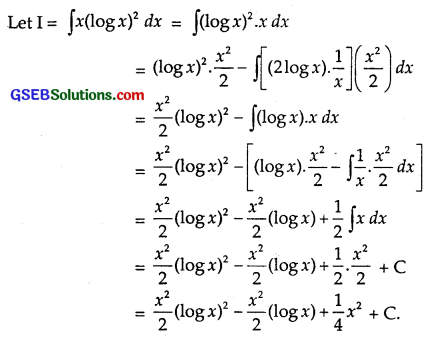Question 15.
(x2 + 1) log x
Solution: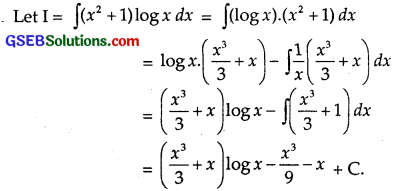Question 16.
ex(sin x + cos x)
Solution:
Let I = ∫ex(sin x + cos x) dx.
Put exsin x = t so that (ex.cos x + sin x.ex)dx = dt.
⇒ ex(sin x + cos x) dx = dt
∴ I = ∫dt = t + C = exsinx + C.Question 17.
$$\frac{x e^{x}}{(1+x)^{2}}$$
Solution: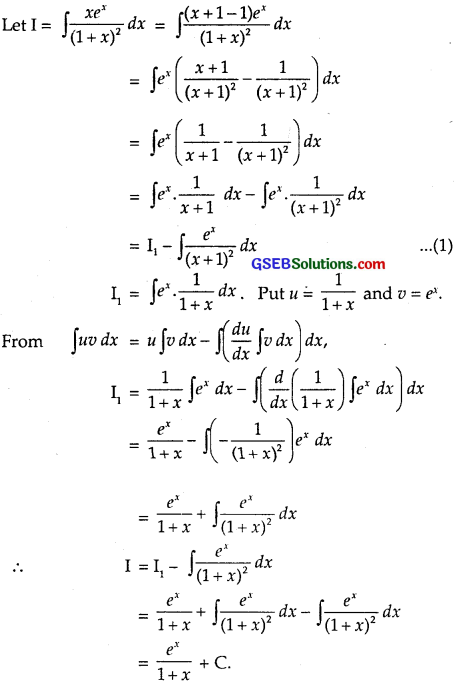Question 18.
ex($$\frac{1+sinx}{1+cosx}$$)
Solution: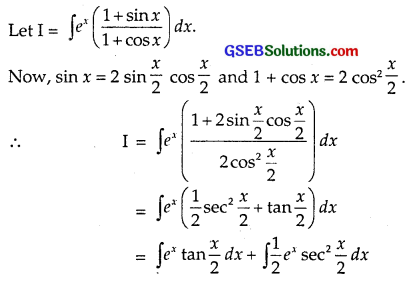Taking tan $$\frac{x}{2}$$ as I function and ex as II function,
integrating ∫ex tan $$\frac{x}{2}$$ dx by parts, we get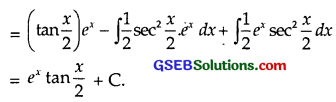Question 19.
ex($$\frac{1}{x}$$ – $$\frac{1}{x^{2}}$$)
Solution: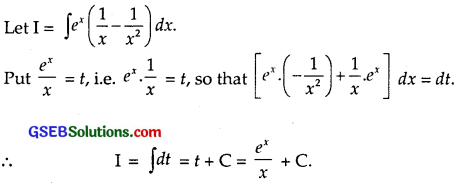Question 20.
$$\frac{(x-3) e^{x}}{(x-1)^{3}}$$
Solution: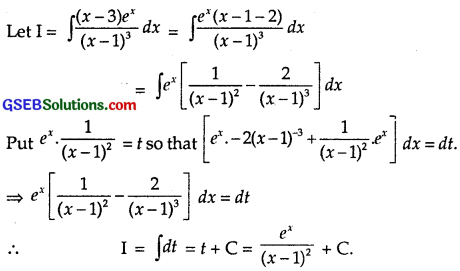Question 21.
e2xsinx
Solution: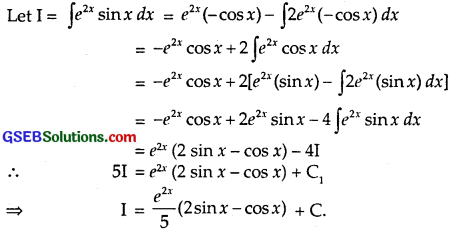Question 22.
sin-1($$\frac{2 x}{1+x^{2}}$$)
Solution: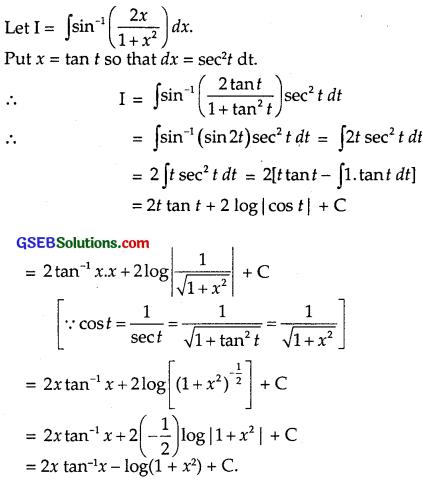Choose the correct answers in the following questions from 23 and 24:
Question 23.
∫ x2 ex3 dx equals
(A) $$\frac{1}{3}$$ ex3 + C
(B) $$\frac{1}{3}$$ ex2 + C
(C) $$\frac{1}{2}$$ ex3 + C
(D) $$\frac{1}{2}$$ ex2 + C
Solution: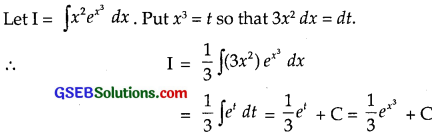∴ Part (A) is the correct answer.Question 24.
∫ ex secx(1 + tan x) dx equals
(A) ex cosx + C
(B) ex secx + C
(C) ex sinx + C
(D) ex tanx + C
Solution: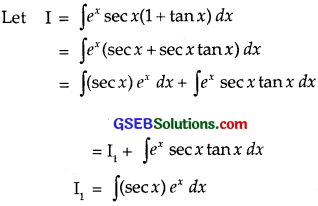Taking sec x as I function and ex as II function, integrating by parts
I1 = (sec x) ∫ex dx – ∫(sec x tan x ∫ex dx)dx
= (sec x)ex – ∫ex sec x tan x dx
Putting this value in (1), we get
I = I1 + ∫ex sec x tan x dx
= (sec x)ex – ∫ex sec x tan x dx
Putting this value in (1), we get
I = I1 + ∫ex sec x tan x dx
= (sec x)ex – ∫ex sec x tan x dx + ∫ex sec x tan x dx + C
= ex sec x + C.
∴ Part (B) is the correct answer.